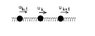# Oscilations of a linear chain of masses and springs

• jncarter

## Homework Statement

A linear chain consists of N identical particles of mass m are connected by N+1 identical, massless springs with force constant k. The endpoints are fixed to walls on each side. In the static configuration each spring is stretched from its relaxed length l0 to a new length l. Construct the Lagrangian for general small oscillations in a plane and show that the transverse and longitudinal modes decouple. Discuss how the frequencies depend on l-l0. Discuss and interpret the behavior for l<l0.

## Homework Equations

$T = \frac{1}{2} \Sigma m_{\alpha} v^{2}_{\alpha}$
$U = \frac{1}{2}\textit{k}(\textit{l}-\textit{l}_{0})^{2}$ I think
L = T - U

## The Attempt at a Solution

I'm having a hard time deciding on the best way to define my coordinates and how they all relate to each other. I know that once I have that figured out I must assume that the x and y displacements are small and use a Taylor expansion. If there are no cross terms or x and y, then the longitudinal and transverse waves must be decoupled. I think I just need the right picture to be drawn.

Thanks for all of the help!

Hi, jncarter!

Think over the equation for the potential. While vibrating, are all springs of equal length in the chain?
For the potential energy, you need the change of length of all springs in terms of the small displacements. What is the change of length of the k-th spring if the k-th particle at the right end displaces by uk, and the (k-1)-th particle at the left end displaces by uk-1 ?

ehild

#### Attachments

•springsmasses.JPG
4.4 KB · Views: 626
My problem is that the springs can be stretched in a plane. I could do it if it were a linear problem. I gave it some more thought though, especially about the potential. To start let $\vec{r}_{n}$ be the location of the nth particle after the displacement. Then
$U_{n} = \frac{1}{2}k(\vec{r}_{n+1} - \vec{r}_{n} - l_{0})^{2} + \frac{1}{2}k(\vec{r}_{n} - \vec{r}_{n-1} - l_{0})^{2}$​
Now using some scribbled drawings I have concluded that
$\vec{r}_{n}^{2} = (l_{0} + u_{n+1}^{x} - u_{n}^{x})^{2} + (y_{n+1}-y_{n})^{2}$​
How does all of that look? I would end up having to sum over the n's and I bet some of the terms will cancel before I even get to considering that the u's and y's are small.

#### Attachments

• spring.bmp
24.9 KB · Views: 641
Fetter and Walecka, Chapter 4, problem 4.11? Me too, buddy.

I'm having trouble with this as well because the problem is kind of unclear to me. It says "Each spring is stretched to l"... does that mean l is the same for each spring? Or each spring just has its own l it's stretched to?

It would be kind of strange if each were stretched to the exact same length. That would really only allow certain starting configurations. Also, every spring can't be stretched to an l<l_0 because...well, because some springs would have to stretch for others to contract.

All springs are identical, having original length l0 and stretched to l at equilibrium. When the k-th particle is displaced from its equilibrium position by (xk,yk), the square of the new length of the k-th spring becomes

$$\sqrt{(l+x_k-x_{k-1})^2+(y_k-y_{k-1})^2}$$.

The k-th spring contributes to the potential energy by

$$U_k=\frac{k}{2}(\sqrt{(l+x_k-x_{k-1})^2+(y_k-y_{k-1})^2}-l_0)^2$$

The potential energy of the system is equal to the sum of the energies of the individual springs. (Jncarter, your formula for the particles will result twice the potential energy.) Put the sum of Uk into the Lagrangian as it is, and linearise after differentiation. Note that x0=0, y0=0 in the first term and xn+1=0, yn+1=0 in the last one.

ehild

Last edited:
Okay, I can see why my potential would be double what it should be. Why is it that there is no subtraction of the neighboring particle in the y-component of the stretched length like there is in the x-component? I'm pretty sure there should be because the triangle I formed for the new length would have a height that depends on the vertical displacement of neighboring particles. See the attached picture. The red arrow points to what I am talking about.

#### Attachments

• spring.bmp
24.9 KB · Views: 680
jncarter said:
Okay, I can see why my potential would be double what it should be. Why is it that there is no subtraction of the neighboring particle in the y-component of the stretched length like there is in the x-component? I'm pretty sure there should be because the triangle I formed for the new length would have a height that depends on the vertical displacement of neighboring particles. See the attached picture. The red arrow points to what I am talking about.
Sorry, I just forgot to type in the other y component. Of course, the difference of the y coordinates of both ends appear. I edited my previous post. Is it correct now?

ehild

Looks good to me. I thought that was what had happened. Otherwise I would have to seriously re-think my geometry ability...

Thank you for taking the time to help me out with this.